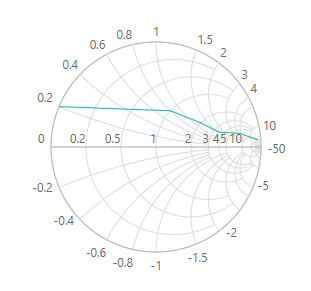Search results

# Dimensions in Blazor Smith Chart component

08 Apr 2021 / 3 minutes to read

You can set the Smith Chart dimensions in the following ways.

• Size using CSS
• Size using API

## Size using CSS

To set the size using CSS, add `ID` to the `SfSmithchart` tag and specify the width and height of the Smith Chart using CSS as shown below.

``````<SfSmithchart ID="dimension">
<SmithchartSeriesCollection>
<SmithchartSeries Points='FirstTransmissionData'></SmithchartSeries>
</SmithchartSeriesCollection>
</SfSmithchart>

<style>
#dimension {
height:300px;
width:300px;
}
</style>

@code {
public class SmithDataSource
{
public double? resistance;
public double? reactance;
};
private List<SmithDataSource> FirstTransmissionData = new List<SmithDataSource> {
new SmithDataSource { resistance= 10, reactance= 25 },
new SmithDataSource { resistance= 6, reactance= 4.5 },
new SmithDataSource { resistance= 3.5, reactance= 1.6 },
new SmithDataSource { resistance= 2, reactance= 1.2 },
new SmithDataSource { resistance= 1, reactance= 0.8 },
new SmithDataSource { resistance= 0, reactance= 0.2 }
};
}``````## Size using API

You can also set size for Smith Chart directly through `Width` and `Height` properties. Using this properties, you can directly mention the width and height of the Smith Chart in pixels or you can set the width and height in percentage.

### In Pixel

In Smith Chart’s `Width` and `Height` property, you can directly give values in pixels like below demonstration. This will render Smith Chart in same size as you mentioned in you code.

``````<SfSmithchart Height="300px" Width="300px">
<SmithchartSeriesCollection>
<SmithchartSeries Points='FirstTransmissionData'></SmithchartSeries>
</SmithchartSeriesCollection>
</SfSmithchart>

@code {
public class SmithDataSource
{
public double? resistance;
public double? reactance;
};
private List<SmithDataSource> FirstTransmissionData = new List<SmithDataSource> {
new SmithDataSource { resistance= 10, reactance= 25 },
new SmithDataSource { resistance= 6, reactance= 4.5 },
new SmithDataSource { resistance= 3.5, reactance= 1.6 },
new SmithDataSource { resistance= 2, reactance= 1.2 },
new SmithDataSource { resistance= 1, reactance= 0.8 },
new SmithDataSource { resistance= 0, reactance= 0.2 }
};
}``````### In percentage

In Smith Chart’s Width and Height property, you can directly give values in percentage like below demonstration, then Smith Chart will be rendered as per the percentage of it’s container size.

``````<div style="height:600px; width:600px">
<SfSmithchart Height="50%" Width="50%">
<SmithchartSeriesCollection>
<SmithchartSeries Points='FirstTransmissionData'></SmithchartSeries>
</SmithchartSeriesCollection>
</SfSmithchart>
</div>

@code {
public class SmithDataSource
{
public double? resistance;
public double? reactance;
};
private List<SmithDataSource> FirstTransmissionData = new List<SmithDataSource> {
new SmithDataSource { resistance= 10, reactance= 25 },
new SmithDataSource { resistance= 6, reactance= 4.5 },
new SmithDataSource { resistance= 3.5, reactance= 1.6 },
new SmithDataSource { resistance= 2, reactance= 1.2 },
new SmithDataSource { resistance= 1, reactance= 0.8 },
new SmithDataSource { resistance= 0, reactance= 0.2 }
};
}``````Contents
Contents